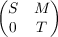#### Vol. 60, No. 1, 1975

 Download this articleFor screen For printingRecent Issues Vol. 325: 1  2 Vol. 324: 1  2 Vol. 323: 1  2 Vol. 322: 1  2 Vol. 321: 1  2 Vol. 320: 1  2 Vol. 319: 1  2 Vol. 318: 1  2Online Archive Volume: Issue:The Journal Subscriptions Editorial Board Officers Contacts Submission Guidelines Submission Form Policies for Authors ISSN: 1945-5844 (e-only) ISSN: 0030-8730 (print) Special Issues Author Index To Appear Other MSP Journals
Rings with quasi-projective left ideals

### Surender Kumar Jain and Surjeet Singh

Vol. 60 (1975), No. 1, 169–181
##### Abstract

A ring R is a left qp-ring if each of its left ideals is quasi-projective as a left R-module in the sense of Wu and Jans. The following results giving the structure of left qp-rings are obtained. Throughout R is a perfect ring with radical N: (1) Let R be local. Then R is a left qp-ring iff N2 = (0) or R is a principal left ideal ring with dcc on left ideals, (2) If R is a left qp-ring and T is the sum of all those indecomposable left ideals of R which are not projective, then T is an ideal of R and N = T L, L is a left ideal of R such that every left subideal of L is projective, R∕T is hereditary, and R is heredity iff T = (0). (3) If R is left qp-ring then R =, where S is hereditary, T is a direct sum of finitely many local qp-rings and M is a (S,T)-bimodule. (4) A perfect left qp-ring is semi-primary. (5) Let R be an indecomposable ring such that it admits a faithful projective injective left module. Then R is a left qp-ring iff R is a local principal left ideal ring or R is a left-hereditary ring with dcc on left ideals. (6) Let R be an indecomposable QF-ring. Then R is a left qp-ring if each homomorphic image of R is a q-ring (each one-sided ideal is quasi-injective). (7) If a left ideal A of left qp-ring R is not projective then the projective dimension of A is infinite, thus lgl.dimR = 0,1, or . An example of a left artinian left qp-ring which is not right qp-ring is also given.

Primary: 16A50
##### Milestones